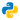# Intro to Seaborn

## Use Seaborn to create a bar graph

Seaborn is a Python visualization library based on matplotlib. It provides a high-level interface for drawing attractive statistical graphics.

I prefer Seaborn due to its wonderful color schemes cough and close integration with pandas, but there are other Python visualization tools. You can see the comparison here.

Let's begin! I will be using a CSV I created for this tutorial:

Patients and the number of their stays at Arkham Mental Asylum

``````import pandas as pd
import seaborn as sns
import matplotlib.pyplot as plt

# Read the csv using pandas module

# Sort by Number of Stays column DESC
budget = budget.sort_values(by='Number of Stays', ascending=False)

sns.set_style("darkgrid")

# Create a bar graph
bar_plot = sns.barplot(x=budget["Name"],y=budget["Number of Stays"], palette="deep")

# Set title of the bar graph
bar_plot.set_title("Arkham's Patients", size=30, color="r", alpha=0.5)

# Set x-axis of the bar graph
bar_plot.set_xlabel("Patients", size =16, color="r", alpha=0.5)

# Set y-axis of the bar graph
bar_plot.set_ylabel("# of Stays", size=16, color="r", alpha=0.5)

# Saves the bar graph as png
plt.savefig("output.png")

# Show the bar graph
plt.show()``````

Ta-da. You can see the result below.

Done! 🙂

## Reference

Seaborn: statistical data visualization